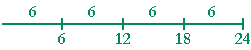THE MEANING OF DIVISION

PROBLEMS

11.   a)  What number times 100 will be 287?  Answer using the division
1.   a)   sign ÷ .

Do the problem yourself first!

287 ÷ 100 = 2.87

"287 divided by 100 equals (or is) 2.87."

11.   c)  What is 287 called?   The dividend.

11.   d)  What is 100 called?  The divisor.

11.   e)  What is 2.87 called?  The quotient.

11.   f)  Prove your answer to part a).    2.87 × 100 = 287

12.   Practice the following.
 3 × 6 = 18. 4 × 8 = 32. 5 × 9 = 45. 6 × 9 = 54. 7 × 6 = 42. 8 × 6 = 48. 9 × 3 = 27. 3 × 8 = 24. 4 × 6 = 24. 5 × 6 = 30. 6 × 5 = 30. 7 × 4 = 28. 8 × 9 = 72. 9 × 6 = 54. 3 × 9 = 27. 4 × 9 = 36. 5 × 7 = 35. 6 × 7 = 42. 7 × 3 = 21. 8 × 5 = 40. 3 × 4 = 12. 4 × 4 = 16. 6 × 3 = 18. 4 × 5 = 20 9 × 6 = 54.

13.   a)  Let this straight line represent 24, and illustrate 24 ÷ 4.13.   b)  Prove   24 ÷ 4 = 6.   6 × 4 = 24

13.   c)  Illustrate 24 ÷ 6.13.   d)  Prove   24 ÷ 6 = 4.   4 × 6 = 24

14.   a)  How many times could you subtract 8 from 40?  5 times.

13.   b)  Write that problem using the division sign ÷ .

40 ÷ 8 = 5

14.   c)  Write that problem using the division bar.

 40 8 = 5

14   d)  In that form, what is 40 called?   The dividend.

14.   e)  What is 8 called?  The divisor.

14.   f)  What is 5 called?  The quotient.

15.   a)  From a bottle that contains 48 oz, how many times could you fill
14.   a)  a 6 oz glass?  8 times.  48 ÷ 6 = 8.

15.   b)  From a bottle that contains 2 quarts, how many times could you
14.  b)   fill an 8 oz glass?  (1 quart = 32 oz)

8 times.  64 oz ÷ 8 oz = 8.

To divide, the units must be of the same kind.

16.   A farmer has a field that is 100 yards long, and he wants to put a
16.   fence post every 4 feet.  How many fence posts must he put?

How many times is 4 feet contained in 100 yards? To answer, we have to change yards to feet because, again, the units must be the same.

Since 1 yard = 3 feet, then 100 yards = 300 feet.  How many 4's are there in 300?

Now, one hundred is made up of twenty-five 4's:  100 = 25 × 4. Therefore, three hundred = (3 × 25) × 4 = 75 × 4. The farmer must put 75 fence posts.

(Alternatively, to divide a number by 4 is equivalent to taking its fourth part. And to take the fourth part, we can take half of half.  Lesson 16, Question 9.  Half of 300 is 150. Half of 150 is 75.)

But what about the fence post at the beginning of the field -- at the "zero" point? In reality, then, he would put 76 fence posts!

17.   If you divide a number by 8, what are the possible remainders?

1, 2, 3, 4, 5, 6, 7.

18.   Divide each number by 8 mentally.  Write the whole number quotient
16.  and the remainder.

17  18  2 R 2.     29  3 R 5.     39  4 R 7.     54  6 R 6.     76  9 R 4.

19.   Divide each number by 7 mentally.  Write the whole number quotient
19.   and the remainder.

19.   17  2 R 3.     25  3 R 4.     31  4 R 3.     41  5 R 6.     61  8 R 5.

10.   Divide each number by 9 mentally.  Write the whole number quotient
10.   and the remainder.

10.   17  1 R 8.     25  2 R 7.     31  3 R 4.     41  4 R 5.     62  6 R 8.

11.   Prove:  157 ÷ 14 = 11 R 3

11 × 14 = 140 + 14 = 154, plus 3 is 157.

12.   How will 436 change if you multiply it by 10 and then divide the
12.   product by 10?

It will not change!

13.   Divide.

 a) 40 5 = 8 b) 35 7 = 5 c) 28 4 = 7 d) 63 9 = 7 e) 54 6 = 9 f) 24 4 = 6 g) 72 9 = 8 h) 27 3 = 9 i) 35 7 = 5 j) 45 9 = 5 k) 32 8 = 4 l) 48 6 = 8
 m) 240  6 = 40 n) 420  6 = 70 o) 720  8 = 90 p) 120  4 = 30 q) 320  4 = 80 r) 450  5 = 90 s) 480  8 = 60 t) 400  5 = 80 u) 540  9 = 60 v) 300  6 = 50 w) 630  7 = 90 x) 360  9 = 40 y) 2100   3 = 700 z) 6400   8 = 800 a') 4200   7 = 600 b') 1000   5 = 200 c') 2000   5 = 400 d') 3000   5 = 600 e') 2000   4 = 500 f') 4000   5 = 800
 14. a) 245 10 = 24.5 b) 245100 = 2.45 c) 245 1000 = .245 d) 9 10 = .9 e) 9  100 = .09 f) 9   1000 = .009

Continue on to the next section.